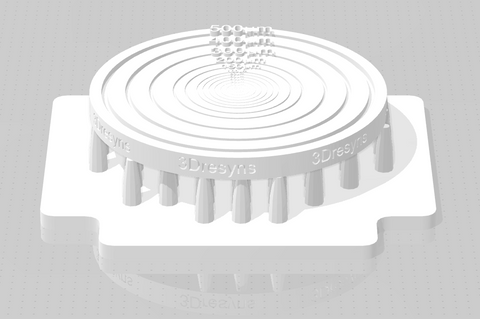Resolution refers to the range of detail or minimum feature size which can be printed with detail. As shown on the surface of the coin the smallest feature size  can be easily evaluated by reading the smallest concentric circle line on the surface of the printed coin, since each circle line has engraved its width and depth in decreasing order from 500 to 2 microns. On the other hand, precision refers to the capability to repeatedly print or "reproduce" the same dimensions of prints over and over again with certain variation. Keep in mind that extremely precise prints are not necessarily dimensionally accurate unless the tree spatial xyz dimensions of prints coincide with the three spatial xyz dimensions of the designed stl files which define the theoretical dimensions of prints as defined in the stl files.

Dimensional accuracy of 3D prints refers to how close the three spatial xyz dimensions of 3D prints are to the theoretical three spatial xyz dimensions of designed prints as defined in the stl file before printing. The stl file of 3Dresyns flat coin has 2 mm thickness (z axis) and 30 mm diameter (xy axis). The relative dimensional accuracy in the xyz axis can be easily measured by measuring the real diameter (xy axis) and thickness (z axis) of the horizontally positioned flat coin. For example, if the thickness of the printed coin is 2.1 mm.  The difference between the theoretical and real value is 0.1 mm and the % difference is 0.1/2x100=5% which indicates the relative dimensional accuracy in the z axis. The same principle applies to the xy dimensional accuracy. If for example the diameter of the printed coin is 30.3 mm.  The difference between the theoretical and real value is 0.3 mm and the % difference is 0.3/30x100=1% which indicates the relative dimensional accuracy in the xy axis.